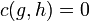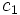# Symmetric and cyclicity-preserving 2-cocycle implies 2-coboundary

Jump to: navigation, search

## Statement

### In cocycle and coboundary language at the origin

Suppose$G$ is a finitely generated abelian group and$A$ is an abelian group. Suppose$c$ is a 2-cocycle for trivial group action of$G$ on$A$ satisfying the following two conditions:

•$c$ is a symmetric 2-cocycle for trivial group action, i.e.,$c(g,h) = c(h,g)$ for all$g,h \in G$.
•$c$ is a cyclicity-preserving 2-cocycle for trivial group action, i.e.,$c(g,h) = 0$ if$\langle g,h \rangle$ is a cyclic group.

Then,$c$ is a 2-coboundary for trivial group action.

### In group extensions language at the origin

Suppose$E$ is an abelian group with a subgroup$A$ and quotient group$E/A \cong G$. Suppose$G$ is finitely generated. Suppose there exists a 1-closed transversal$T$ of$A$ in$E$ (i.e., a collection of coset representatives, in other words,$T$ intersects each coset at exactly one point, with the property that any power of an element in$T$ is also in$T$). Then, in fact,$A$ is a direct factor of$E$ and in particular we can write$E$ as an internal direct product$A \times G$.

### In cocycle and coboundary language away from the origin

Suppose$G$ is a finitely generated abelian group and$A$ is an abelian group. Suppose$c_1$ and$c_2$ are 2-cocycles for the action of$G$ on$A$ such that$\operatorname{Skew}(c_1) = \operatorname{Skew}(c_2)$. Then, if$c_1$ and$c_2$ are both cyclicity-preserving, we must have$c_1 = c_2$.

## Proof

### Proof in group extensions language at the origin

PLACEHOLDER FOR INFORMATION TO BE FILLED IN: [SHOW MORE]